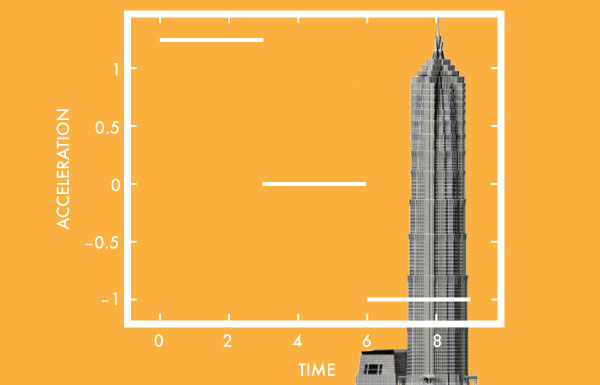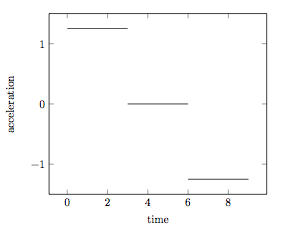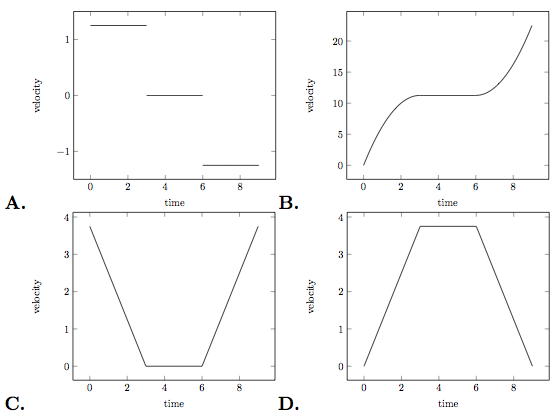# Shanghai Tower

Calculus Level 1The Shanghai Tower has an architectural height of 632 meters, and is equipped with the world's fastest elevator which can travel at $18$ m/s! If we assume that it travels at it's maximum speed, then it would take just under 36 seconds to reach the top. However, this assumption is unrealistic, as the elevator needs time to increase its velocity, i.e. accelerate.

If the acceleration of the elevator is given by the following graph:Which of the following is the best graph to illustrate the velocity of the elevator?×

Problem Loading...

Note Loading...

Set Loading...